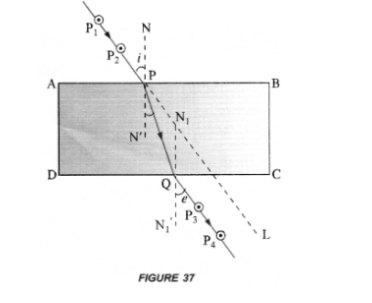# Why does a light ray incident on a rectangular

Question:

Why does a light ray incident on a rectangular glass slab immersed in any medium emerges parallel to itself? Explain using a diagram.

Solution:

Perform an experiment to demonstrate that light bends from its path, when it falls obliquely on the surface of a glass slab. Also show that angle of

incidence is about equal to the emergent angle.

1. Take a glass slab and place it on a white sheet of paper fixed on a drawing board.
2. Mark the boundary ABCD of the glass slab.
3. Fix two pins P1 and P2, vertically on the drawing board such that line joining the pins is inclined to the edge AB of the glass slab (Figure 37).4. Now, look through the glass slab from opposite side so that the images of pins $P_{1}$ and $P_{2}$ are seen exactly in line. Fix two pins $P_{3}$ and $P_{4}$, vertically on the drawing board such that pins $P_{3}, P_{4}$ and image of $P_{1}$ and $P_{2}$ are exactly in one line.

5. Remove the glass slab and all pins. Join the points $P_{1}$ and $P_{2}$ with a line and extend this line to touch the edge $A B$ at $P$. Similarly join the points $P_{3}$ and $P_{4}$ with a line and extend the line to touch the edge DC at Q.

6. Join points $P$ and $Q$ with a straight line.

7. Draw normal $\mathrm{NN}^{\prime}$ at $\mathrm{P}$ on the edge $\mathrm{AB}$ and a normal $\mathrm{N}_{1} \mathrm{~N}_{1}$ ' at $\mathrm{Q}$ on the edge $\mathrm{DC}$.

8. Measure $\angle P_{2} P N$ and $\angle P_{3} Q N_{1}^{\prime}$ using a protractor or dee.

This experiment shows that when light falls obliquely on a glass slab, it bends along PQ from its original path along PL.

Also ∠P2PN ≈ ∠P3QN1‘. That is, angle of incidence is about equal to the emergent angle.

Note : Distance between pins P1 and P2, and between P3 and P4 must be large.